# Extracting a sub string in Microsoft Excel

In this article we are going to learn what the sub string functions are and how we can get sub-string in Microsoft Excel.

Sometimes, we may need to extract some text from a string.  And to do so there are so many sub-string excel formula, from which we will use the RIGHT,  LEFT,  SEARCH,  LEN,  FIND, ISNUMBER  and  IF formulas in Microsoft Excel.

To extract the excel substring from a string follow the below given steps:-

Example 1: We have a list of names in Column “A” and we need to extract the First name from the list.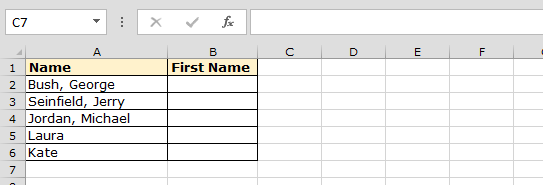• Select the cell B2.
• Enter the formula=IF (ISNUMBER (FIND (",",A2)),RIGHT(A2,LEN(A2)-FIND(",",A2)-1),A2)
• Press Enter on the keyboard
• Thisfunction will return the first name from the cell A2
• To copy the formula to all the cells, copy the formula cell, press the keys“CTRL + C” and select the cell B3 to B6 and press keys“CTRL + V”on the keyboard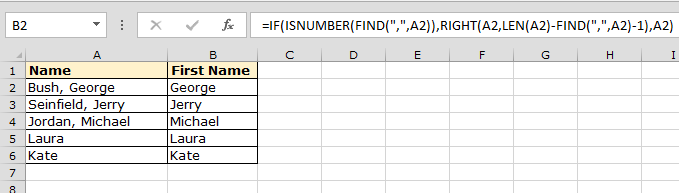Let’s take an another example to extract the sub strings from the Left

Example 2:- We have list of email ids from which we need to extra only the name which is all the text before the “@” symbol.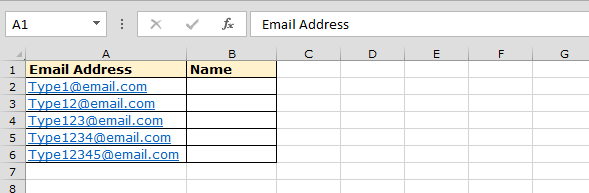This means that the “@” symbol is located at the 5 position in the text string, starting from the left-most character.

To separate the name from the list by using the “Left” function along with “Search” function  follow the below mentioned steps:-

• Select the Cell B2, write the formula=LEFT (A2, SEARCH ("@", A2)-1)
• Press Enter on the keyboard
• The function will return the name – Type1 as per the email Type1@email.com
• To copy the formula to all the cells press key “CTRL + C” on the keyboard and select the range B3 to B6 and press keys“CTRL + V”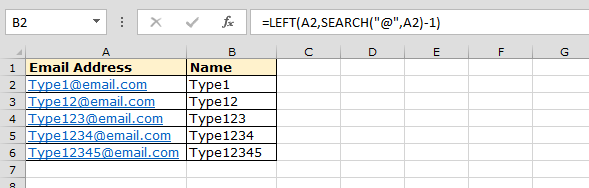Let’s take an another example to extract the sub strings from the Middle

Example 3: We have a list of Names in Column “A” and we need to pick the middle name from the list.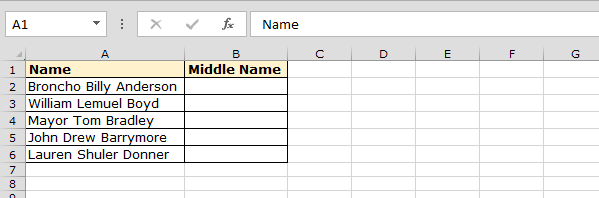To pick the Middle name from the list, we will use the MID function along with the SEARCH function.

• Select the cell B2
• Enter the formula  =MID(A2,SEARCH(" ",A2,1)+1,SEARCH(" ",A2,SEARCH(" ",A2,1)+1)-SEARCH(" ",A2,2)) and press Enter on the keyboard
• It will return the middle name from the cell A2
• To copy the formula in all cells press key “CTRL + C” and select the cell B3 to B6 and press key “CTRL + V”on your keyboard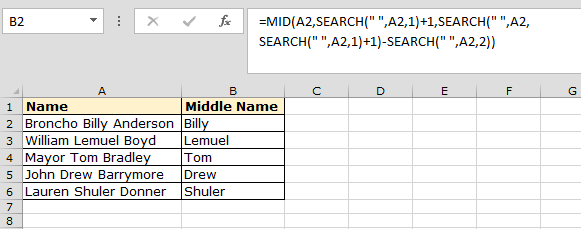This is the way you can extract the sub string from a string by using the above mentioned formulas in Microsoft Excel.We would love to hear from you, do let us know how we can improve, complement or innovate our work and make it better for you. Write us at info@exceltip.com

1.2.